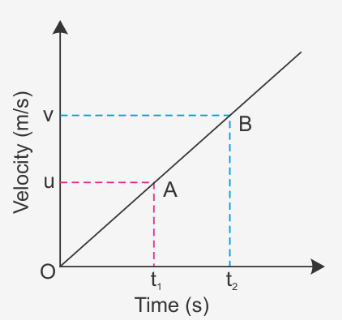# Derive the first equation of motion v = u + at, using the velocity - time graph for a body

Derive the first equation of motion v = u + at, using the velocity-time graph for a body moving along a straight line with uniform acceleration.

Consider a velocity-time graph for a uniformly accelerated body starting from rest is represented as follows.u: velocity at time t1
v: velocity at time t2

If acceleration is represented as a ,then, acceleration is defined as the rate of change in velocity.This is first equation of linear motion.